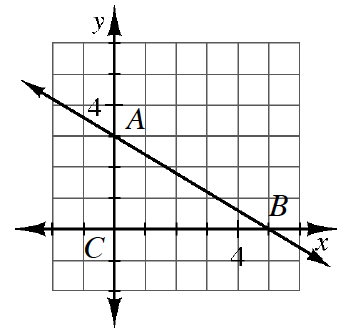### Home > GC > Chapter 3 > Lesson 3.1.3 > Problem3-30

3-30.Examine the graph of line $\overleftrightarrow{AB}$ at right.  3-30 HW eTool(Desmos)

1. Find the equation of $\overleftrightarrow{AB}$.

Identify the slope and y-intercept from the graph and use the equation $y=mx+b$ to write the equation of the line.

2. Find the area and perimeter of $ΔABC$.

The area of a triangle is $(0.5)(\text{height})(\text{base})$.
Find the length of the hypotenuse by using the given leg lengths and the Pythagorean Theorem.

3. Write an equation of the line through $A$ that is perpendicular to $\overleftrightarrow{AB}$.

The slope of a perpendicular line is negative the reciprocal of the original line.